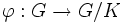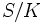# Difference between revisions of "Exactly n elements of order dividing n in a finite solvable group implies the elements form a subgroup"

## Statement

Suppose$G$ is a Finite solvable group (?), and$n$ is a natural number dividing the order of$G$. If there are exactly$n$ elements of$G$ whose$n^{th}$ power is the identity element, then these elements form a subgroup. This subgroup is clearly a normal subgroup. In fact, it is a characteristic subgroup, and even better, is a fully characteristic subgroup.

## Proof

Given: A group$G$. A natural number$n$.$S$ is the set of$n^{th}$ roots of unity in$G$ (i.e., the set of elements of order dividing$n$, and$S$ has$n$ elements.

To prove:$S$ is a subgroup.

Proof: By fact (1),$G$ has a nontrivial elementary abelian normal subgroup$K$ of order$p^i$ for some prime power$p^i$ dividing the order of$G$. We split the proof into two cases, based on whether$p$ divides$n$ or not.

### Case that$p$ divides$n$

In this case,$K \subseteq S$. Let$n = p^jm$ and$|G| = g = p^kl$ where$m,l$ are relatively prime to$p$. Clearly,$j \le k$ and$m|l$.

1. Let$u = p^{\max(j-i,0)}m$. Then, the order of$G/K$ is divisible by$u$: This is clear from the fact that the order of$G/K$ equals$p^{k-i}l$, and since$m|l$ and$j \le k$, this follows.
2.$up$ divides$n$: This follows essentially from the fact that exponent of$p$ in$u$ is strictly less than that in$n$.
3. There exists some natural number$r$ such that$G/K$ has$ru$ elements of order dividing$u$: This follows from fact (2).
4. Let$\varphi:G \to G/K$ be the quotient map. The set of elements$x \in G$ such that$\varphi(x)^u = 1$ has size$rup^i$: This follows from step (3).
5. If$\varphi(x)^u = 1$, we have$x^{up} = 1$: This follows from the fact that the order of any element in$K$ is either$1$ or$p$.
6. If$\varphi(x)^u = 1$, we have$x^n = 1$: This follows from the previous step and step (2), which says that$up|n$.
7.$r = 1$,$j \ge i$ and$x^n = 1 \iff x^u \in K$: By steps (3) and (5), there are at least$rup^i$ elements$x$ for which$x^n = 1$. Thus,$rup^i \le n$. Simplifying, we obtain that $\max(i,j)\$ . This forces$r = 1$ and$j \ge i$. Further, since equality holds even under these assumptions, we conclude that the$rup^i$ solutions to$x^u \in K$ exhaust all solutions to$x^n = 1$.
8. The set of elements$y \in G/K$ such that$y^u = 1$ forms a subgroup. In particular,$S/K$ is a subgroup: By the previous step,$r = 1$, so there are exactly$u$ solutions in$G/K$ to$y^u = 1$. By fact (3),$G/K$ is solvable, so the induction hypothesis applies, and the set of solutions forms a subgroup.
9.$S$ is a subgroup: It is the inverse image in$G$ of a subgroup of$G/K$.